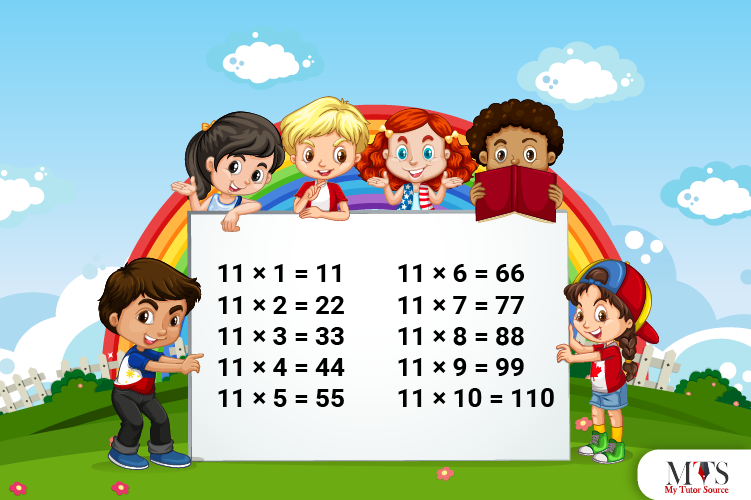# Multiplication Table of 11 – Tips to Memorize 11 Times Table & Example QuestionsMultiplication tables come in handy, whether you are solving trigonometry, geometry, or algebra or complex calculations like number theory, calculus, or theorems. It’s the responsibility of every school teacher or math tutor to help students memorize multiplication tables 1 – 20. Learning multiplication is important as not all exams allow calculators. Let us learn 11 times table, a method to read it, and a few tips and examples to memorize it on fingertips.

11 x 1 = 11
11 x 2 = 22
11 x 3 = 33
11 x 4 = 44
11 x 5 = 55
11 x 6 = 66
11 x 7 = 77
11 x 8 = 88
11 x 9 = 99
11 x 10 = 110
11 x 11 = 121
11 x 12 = 132
11 x 13 = 143
11 x 14 = 154
11 x 15 = 165
11 x 16 = 176
11 x 17 = 187
11 x 18 = 198
11 x 19 = 109
11 x 20 = 220

## Method to Read Table of 11

This section is designed to help you read the 11 times table. Go through the multiplication table of 11 in the words given below for better learning and understanding.

Eleven ones are eleven (11)
Eleven twos are twenty-two (22)
Eleven threes are thirty-three (33)
Eleven fours are forty-four (44)
Eleven fives are fifty-five (55)
Eleven sixes are sixty-six (66)
Eleven sevenths are seventy-seven (77)
Eleven eighths are eighty-eight (88)
Eleven nines are ninety-nine (99)
Eleven tens are one hundred and ten (110)
Eleven elevens are one hundred and twenty-one (121)
Eleven twelves are one hundred and thirty-two (132)
Eleven thirteenths are one hundred and forty-three (143)
Eleven fourteens are one hundred and fifty-four (154)
Eleven fifteenths are one hundred and sixty-five (165)
Eleven sixteenths are one hundred and seventy-six (176)
Eleven seventeens are one hundred and eighty-seven (187)
Eleven eighteenths are one hundred and ninety-eight (198)
Eleven nineteens are two hundred and nine (209)
Eleven twenties are two hundred and twenty (220)

## Tips for Memorizing Multiplication Table of 11

Here are the two quick tips for memorizing the 11 times table:

1. The pattern of the first 9 multiples of the multiplication table of 11 is the same. It is the single natural number written twice. For example, when you multiply 11 with any natural number, it would be double that natural number, i.e., 11 x 9 = 99
2. Use the repeated addition method. Add 11 into the previous product to find the answer to the next multipliers. For example, to calculate the product of 11 x 4, add 11 into 33, which is the product of 11 x 3. Thus, 33 + 11 = 44.

### Example Question of Table of 11

Question: A team football has 11 players. If the coach will distribute 6 bags to each. How many bags were distributed in total?

Solution:

Number of players = 11
Number of bags per player = 6
Therefore,
Number of bags in total = 11 x 6 = 66
Hence, 66 bags were distributed in total.

Question: Using the 11 times table, find out how many elevens are in 121?

Solution:

Let ‘p’ be the number
p x 11 = 121
p = 121 / 11
p = 11
Hence, the total number of eleven in 121 are 11.

Question: Find a number just after 6 times eleven?

Solution:

6 times eleven = 6 x 11 = 66
The number after = 66+1 = 67
Hence, the number after 6 times eleven is 67

Question: Find a number just before 8 x 11?

Solution:

Using 11 times table = 8 x 11 = 88
The number before = 88 – 1 = 87
Hence, the number before 8 x 11 is 87.

Question: There are 11 boxes with 7 books in each box. How many books are there in all?

Solution:

Given that,
Boxes = 11
Books in each box = 7
Thus,
Total number of books = 11 x 7 = 77
Hence, there are 77 books in all.

Question: Find 11 times 5 plus 8 minus 4?

Solution:

Give that,
11 times 5 plus 8 minus 4
In mathematical form,
= (11 x 5) + (8 – 4)
= 55 + 4
=59
Hence, the 11 times 5 plus 8 minus 4 equals 59.

### Find Top Tutors in Your AreaWith over 3 years of experience in teaching, Chloe is very deeply connected with the topics that talk about the educational and general aspects of a student's life. Her writing has been very helpful for students to gain a better understanding of their academics and personal well-being. I’m also open to any suggestions that you might have! Please reach out to me at chloedaniel402 [at] gmail.com